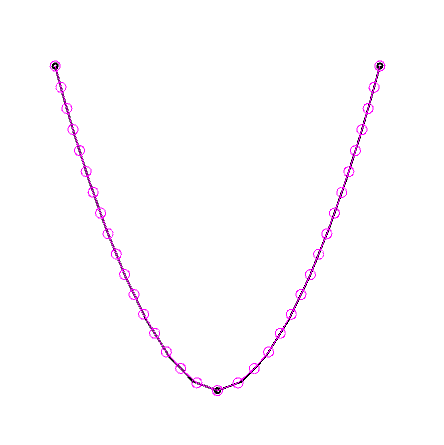# Spiral tests in 3D

This weekend I’m using toxiclibs to create better 3D geometry for my 3D printable spirals, so I won’t have to use any support material and the end result will be a smoother shape that is much faster to print.

I’m using splines (smooth curves) to create geometry and smooth over my blockier, hand-crafted, simply down-sampled geometry.  The result is different, because before I was using the (scaled) average of chunks of audio data and now I am doing some smoothing based on a bit of arbitrary geometry in the form of parameterized curves. This is important to get a final result because trying to directly map the audio onto 3D shapes results in waaaaay to much data for the computer to handle!  Each swept curve profile – the circular ribs of the inner skeleton that form the spiral’s tubular shape  – contained about 389 points to start, at high quality, and there are thousands of those alone, without counting all the triangles they generate!  The meshes either took ages to generate or didn’t generate at all.

To test, I created a simple triangle as the basis for a spline curve, to see how much detail I really needed around the sharp edges of the model.spline resolution of 22 – 36 points

A quality – really, this is called “resolution” in toxiclibs, as in Spline2D.computeVertices(int res) – of 2 results in 389 points for the spline, but a pretty reasonable quality/resolution of 39 results in only 21 points!

```
/*
* 2015 By Evan Raskob: Testing quality of splines on 2D curves.
* quality results in 36 points for a triangular spline.
* quality results in 376 points!
* quality isn't bad - 21 points
*
*
*
*/
import processing.opengl.*;
import toxi.geom.*;
import java.util.Iterator;
import java.util.List;

Spline2D spline;
List<Vec2D> verts, points;
int subDiv = 10;
int quality = 22;

void setup() {
size(800, 800, P3D);
smooth();
cursor(CROSS);

Polygon2D tri = (new Triangle2D(new Vec2D(0, 0), new Vec2D(0.5, 1), new Vec2D(1, 0)))
.toPolygon2D();

for (Vec2D p : tri.vertices) {
println("vert: " + p);
}

spline=new Spline2D(tri.vertices);
spline.updateCoefficients();
//spline.delta.set(points.x*0.75,0,0);
//spline.delta[numP-1].set(-points[numP-2].x,0,0);
spline.computeVertices(subDiv);
verts = spline.getDecimatedVertices(quality, true);
println("quality["+quality+"] results in " + verts.size() + " points");
points = spline.pointList;
}
void draw() {
background(255);
stroke(0);
strokeWeight(2);
noFill();

// draw original
pushMatrix();
translate(width/2, height/2);
beginShape();
Iterator i=spline.vertices.iterator();
while (i.hasNext ()) {
Vec2D v=(Vec2D)i.next();
vertex(v.x, v.y);
}
endShape();

// draw key points
for (Vec2D p : points) {
ellipse(p.x, p.y, 5, 5);
}

strokeWeight(1);
// draw decimated
stroke(255, 0, 255);
beginShape();
i=verts.iterator();
while (i.hasNext ()) {
Vec2D v=(Vec2D)i.next();
vertex(v.x, v.y);
}
endShape();
i=verts.iterator();
while (i.hasNext ()) {
Vec2D v=(Vec2D)i.next();
ellipse(v.x, v.y, 10, 10);
}
popMatrix();

}

void keyPressed()
{
if (key == '-')
{
quality = max(quality - 1, 2);
} else if (key == '+')
{
quality = min(quality + 1, 100);
}

verts = spline.getDecimatedVertices(quality, true);
println("quality["+quality+"] results in " + verts.size() + " points");
}

```

The end result of today was to see just how terrible it would look to use the default (and simple)  ProfilePathAlignment example from toxiclibs to generate a more complex, swept shape, and you can see from the image below that it is pretty terrible, indeed:

That’s some tortured geometry, right there…

The code is in a public gist at: ProfilePathAlignmentSpline2D

I was better off using the Parallel Transport Frames method implemented in the tubesP5 library for Processing, as the Hemesh library (which was looking very cool) has some sort of bug where I’m only getting 1/2 the triangles when I export to STL files (which I need to email wblut about!)

The only issue is that the tubesP5 library sometimes takes ages to create a closed path, probably due to all the try/catch code in it.  Time to either optimize it a bit, or borrow from ThreeJS’s tube geometry class.

There’s also a lot of discussion around Rotation Minimising Frames but I need some more time to digest that paper… my math is a bit rusty for that kind of fun.